# 1.07 Sinusoidal signals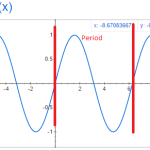The next few sections in The Arts of Electronics deals with signals.  What are the characteristics of signals?  Amplitude, frequency, shape, etc.

The values we are looking at is voltage as a function of time.  The most basic signal is the sinusoidal signal.  Have you ever graphed y=sin(x) and end up with: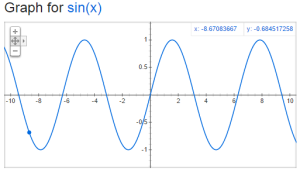But how would you compare this wave to the following: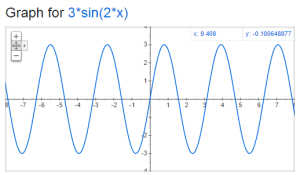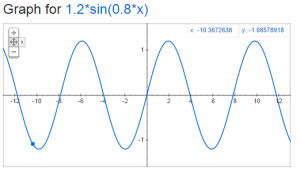There are two key things to think about when comparing sine waves:  frequency and amplitude.    The amplitude is the distance from y=0 to the top of the sine wave.  The frequency is how many times the wave repeats in a given period of time.  We typically use the unit hertz (Hz) for frequency, which is one cycle (or period) per second.  Lets take a look at period and amplitude in our original sine graph:The formula that you should remember is

V=A*sin(2*pi*f*t)

A is the amplitude in volts, f is the frequency in Hz and t is the time in seconds.

# 1.06 Small-signal resistanceOhm’s law is R=V/I.  For the ordinary resistance, this is a constant proportion for a large range of V.  But this is not true for all devices.  Today we look at the Zener diode which has a high resistance up to a point and then almost no resistance for a large range of V.  Also, we look at the tunnel diode (also known as the Esaki diode) which actually has a negative resistance over a particular range of V.

# 1.05 Thevenin’s equivalent circuit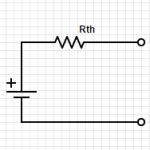Any two terminal network of voltage sources and resistors can be replace by a single voltage source in combination with a single resistor.  This is called the Thevenin’s equivalent circuit: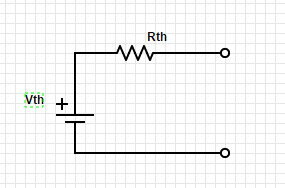The first step is to identify the two terminals of the network.

Then to figure out Rth, replace any voltage sources with short circuits and any current sources with open circuits.

Calculate the voltage between the two terminals.

Then figure out Vth between the two terminals.

To figure this out using testing and measuring, it is only necessary to find the voltage at the two terminals with power on, and the resistance with power off.

This can greatly simplify circuit analysis of complex circuits.

Check out videos on YouTube with more detailed information:

# 1.04 Voltage and current sourcesLet’s take a look at the symbols for voltage sources and current sources. Also, let’s discuss the properties of the ideal voltage source and the ideal current source.

The ideal voltage source will maintain the same voltage between its terminals regardless of the load. This is easiest with an open circuit but becomes impossible with a short circuit.

The ideal current source will maintain the same current regardless of the resistance of the load. This is easy with an open circuit but becomes more and more difficult as the resistance increases.

# 1.03 Voltage Dividers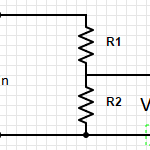Voltage Dividers take an input voltage and gives you a smaller, fractional output voltage. This can be useful for volume control or when interfacing between logic circuits that run at 5V with new/lower power devices that run at 3.3V.

A voltage divider is a simple circuit made up of only two resistors (R1 & R2 in the diagram below).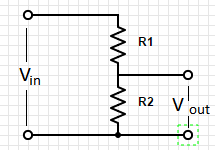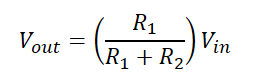# 1.02 Relationship between voltage and current: resistors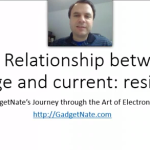Art of Electronics 2nd Edition p 4-7

What you MUST learn

Resistance (represented in formulas as “R”) measured in Ohms which is represented using the symbol: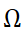Resistance is the relationship between Current (I) and Voltage (R).

This relationship is defined by Ohm’s law:  R = V/I.

Circuit diagrams represent resistors as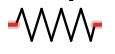Resistors in Series: (Always bigger)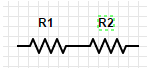R= R1 + R2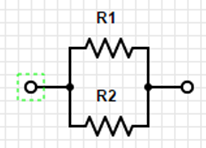Resistors in Parallel: (Always smaller)

R = (R1*R2)/(R1+R2)

Shortcut #1:

A large resistor in series with a small resistor has the resistance of the larger one, roughly.

A large resistor in parallel with a  small resistor has the resistance of the smaller one, roughly.

Try to develop an intuitive sense of the voltage and current in the parts of the circuit.  Do not try to calculate to be extremely precise because the precision of the components will make this pointless.  Also, good circuit design should be tolerant of variations.

Power:

P=IV

Using Ohm’s law you get:

P=(I^2)R

P=(V^2)/R

Things good to learn

The inverse of resistance is conductance (represented by “G” in formulas)  G=1/R

Restatement of Ohm’s law:  I=GV

Conductance is measured in siemenssiemens is also known as mho (ohm spelled backwards)

# 1.01 Voltage and Current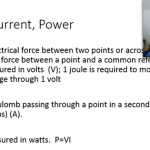Art of Electronics 2nd edition, pages 2-4

Overview

Voltage & Current describe two aspects of electronic circuits that are fundamental to understand and on which everything else will build on.

What you MUST learn

Voltage refers to a force that when applied across a circuit causes current to flow.  Voltage is measured in volts (using the symbol V) also called a “potential difference”.  It is measured across two points in a circuit.  A joule of work is needed to move a coulomb of charge through a potential difference of one volt. A coulomb is the charge of about 6×10^18 electrons.

Current (symbol: I) is the measure of electronics through a point in a circuit.  It is measured in amperes (amps) (symbol: A).  1 ampere (amp) is 1 coulomb of charge passing through a point per second.

Conservation of Charge:  Kirchhoff’s current law

The sum of currents into a point or node is equal to the sum of the currents out of a point or node.  This is referred to as Kirchhoff’s current law.

Kirchhoff’s voltage law

The sum of voltage drops through one branch of a circuit will equal the sum of voltage drops across the other branches of the circuit.

P=VI

Power (work per unit time), measured in watts.  (1 W = 1 J/s)

P=VI = (work/charge) x (charge/time) = work/time

P=VI= (1 joules/1 coulomb) x (1 coulomb/1 second) = 1 joules/second = watt.

Things good to learn

Voltage is usually written with the symbol V but sometimes E is used.   Voltage is also called a “potential difference” or electromotive force (EMF).

Prefixes:

 Multiple Prefix Symbol 10^12 tera T 10^9 giga G 10^6 mega M 10^3 kilo k 10^(-3) milli m 10^(-6) micro µ 10^(-9) nano n 10^(-12) pico p 10^(-15) femto f

When abbreviating a unit with a prefix, the unit follows the multiplier with no space and the unit is capitalized.  However, no capitalization for both prefix and unit when spelled out.  1mW = 1 milliwatt

1 MV = 1 megavolt

Related information and insights from my other books

Introductory Circuit Analysis 9th edition – chapter 2

Structure of the atom:

nucleus is made up of protons (that have a positive charge) and neutrons that have a negative charge.  The proton and neutron are relatively the same mass.  But the electron is considerably smaller (1836 times smaller) than the proton but has a negative charge that is equal to the positive charge of the proton.

Other things of interest

If you need more help

Voltage

Current

Joule

If you wanted to know

Who defined:

Voltage

Volt

Current

amp

joule

watt

multiplier prefixes

People who contributed:

Watt

# Book Review: Ishmael: An Adventure of the Mind and Spirit by Daniel QuinnIshmael by Daniel Quinn is one of the more thoughts provoking books that I have read in a while.   It is a book on philosophy framed as a discussion between an unlikely teacher and a pupil we can all related to.  There are several core ideas presented in the book:

1) Past performance is no guarantee of future returns.  Just because something has survived for many years does not mean that it will continue to do so.   Examples from the early days of flight are given to convince us of this.  Hopeful inventors with machines meant to fly being thrown off of cliffs.  They fly for a while, but regardless of how much effort the pilot expends, they will not stay in the air.  Machines like in this video: www.youtube.com/watch?v=8y3fIr4dVYE

2) The web of life is being trimmed and cut away so that it only supports man’s needs.  If an animal or plant does not help us then it is eliminated.  The robust web is being reduced to a fragile chain that needs only be broken at a few places to cause the entire system to collapse.

The book goes into more details and explains these concepts in more details.   Take a look at Ishmael by Daniel Quinn.

# Simple Tablet Wall Mount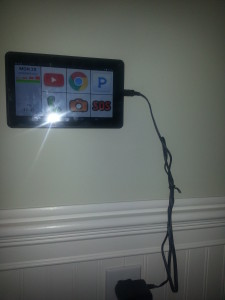We have been slowly collecting tablets in the form of iPad (original), iPad mini, HP slates, and recently through a purchase of 2 RCA slates that we got for \$65 from Walmart during the most recent black Friday sale.

My wife had a good idea of mounting one in the laundry room so that she can listen to music while doing laundry.  We have been thinking of other uses, example: intercom.

There are many options available for mounting.  They make mounts just for this purpose and there are a few really cool DIY ideas.  However, looking for something quick and simple, I decided to go with adhesive valcro tape.  This worked wonder.

I just put two strips on the back, and attached to the wall

# Preparing for my journey through “The Art of Electronics”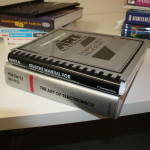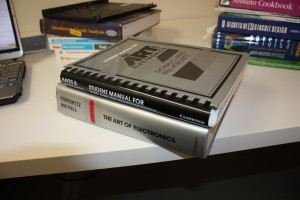“The Art of Electronics” by Horowitz and Hill is the definitive guide to Analog and Digital electronics.  I had bought the 2nd edition over a decade ago, with the intention of going through it.  But this year is going to be the year.  And I am hoping that you will help me.

My plan is to go through a section a day, and to write a short blog post summarizing what I learned.  I have collected several electronics books, and I plan to make reference to them as I go on this journey.  These include:

In addition to a brief summary of what I learn from that section, I plan to create a video post, create additional exercises, and assemble related circuits.  I am hoping that it will be an exciting adventure, and that by having to produce a daily blog post, I will have the discipline to see it through.  And should I stumble, hopefully an encouraging comment or email from you will nudge me back on the path.

First thing is that I need some help by those that are familiar with Electronics.  I am an Amateur with hopes of achieving proficiency.  I do not want my struggles to cause problems for others following along.  Therefore, I would like to have someone knowledgeable review my notes before I publish them on this blog.  If you would be willing to help out, then please email me at nathan.price@gmail.com.  Let me know how much you would be willing to help out.  I figure each blog post would take 15 minutes or so to review.   I would like to give you credit for reviewing, but if you want to remain anonymous, then that is fine too.